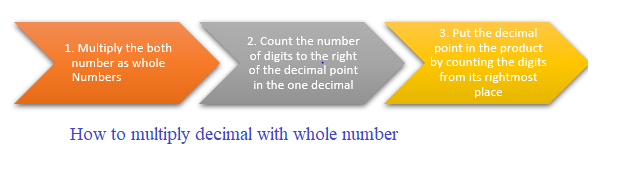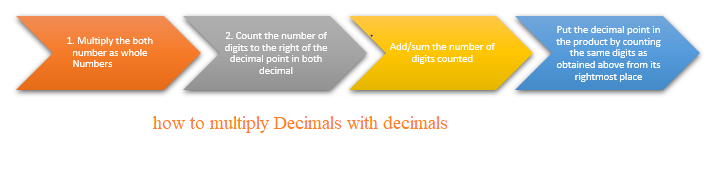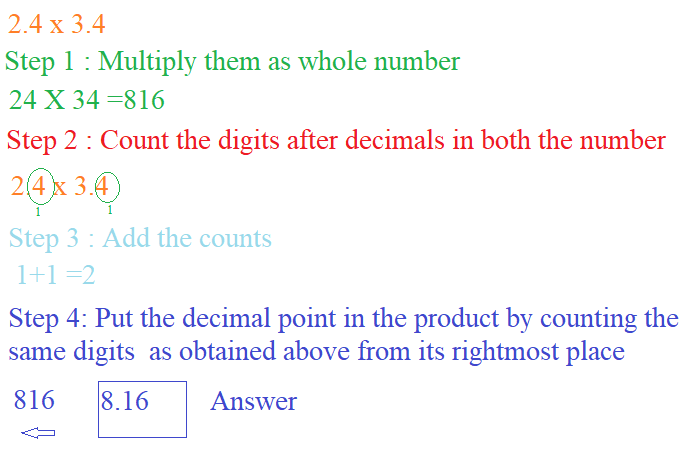# Multiplying Decimals | Multiply Decimals with whole numbers

## Multiplication of a Decimals by a Whole Number

how to multiply Decimals with whole numbers
Here are the steps to multiply Decimals with whole numbers
a. Multiply the both number as whole Numbers
b. Now Count the number of digits to the right of the decimal point in the one decimal
c. Put the decimal point in the product by counting the digits from its rightmost placeLet take the multiplication
$1.2 \times 4$
Step 1: Multiply them as whole number
$12 \times 4 = 48$
Step 2: Count the number of digits to the right of the decimal point in the one decimal
1
Step 3: Final answer is obtained by putting the decimal point in the product by counting the same digits as obtained above from its rightmost place
$4.8$
1. $3 \times 1.5$
$3 \times 1.5= 4.5=$

2. $2 \times 4.4$
$2 \times 4.4= 8.8$

## Multiplication of a Decimals by a decimal

how to multiply Decimals with decimals
Here are the steps to multiply Decimals with decimals
a. Multiply the both number as whole Numbers
b. Now Count the number of digits to the right of the decimal point in both the decimals
c. Add/sum the number of digits counted
c. Put the decimal point in the product by counting the same digits as obtained above from its rightmost placeLet take the multiplication
$1.2 \times 4.2$
Step 1: Multiply them as whole number
$12 \times 42 = 504$
Step 2: Count the number of digits to the right of the decimal point in both decimal
for 1.2 , count is 1 and for 4.2 ,count is 1
step 4: Add/sum the number of digits counted
1+ 1=2
Step 3: Final answer is obtained by putting the decimal point in the product by counting the same digits as obtained above from its rightmost place
$5.04$1. $3.2 \times 1.5$
$3.2 \times 1.5= 4.80=$

2. $2.1 \times 4.4$
$2.1 \times 4.4= 9.24$

### Multiplication of a Decimals by 10,100,1000

Multiplication of a Decimals by 10,100,1000 is pretty easy.The digit in the product remains as same as in the decimal number. Just the decimal point is shifted towards right by the same places as there are zeroes over one
Example
i. $4.23 \times 100$.
Step 1: The digit remains same as in the decimals
4.23
Step 2: There are two zeroes over 1
Step 3: Shift the decimal point towards right by 2 places
4.23 becomes 423

ii.$.23 \times 1000$.
Step 1: The digit in the product remains as same as in the decimal number
.23
Step 2: There are three zeroes over 1
Step 3: Shift the decimal point towards right by 3 places
.23 becomes 230
iii.$1.2 \times 100 = 120 =$

iv.$.0765 \times 10 = .765$
v.$.0145 \times 100 = 1.45$
vi. $1.067 \times 10 = 10.67$

### Quiz Time

Question 1 Which of these decimals is greatest?
A) $.01 \times 10$
B)$.001 \times 100$
C)$.10 \times 10$
D)$1.01 \times 100$
Question 2 $1.67 \times 2.4$ ?
A)$4.008$
B)$40.08$
C)$.4008$
D)$400.8$
Question 3 The value of multiplication $.25 \times 8$
A)$.2$
B)$20$
C)$2$
D)$.02$
Question 4 The value of multiplication $.023 \times 1000$
A)$23$
B) $230$
C) $2.3$
D) $2300$
Question 5 The value of multiplication $.0003 \times 10$?
A)$.003$
B)$.03$
C)$3$
D)$.00003$